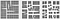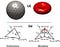# Create Your Own Spring Network Simulation (With Python)

For today’s recreational coding exercise, we will simulate a spring network: an array of nodes connected by springs that obey Hooke’s Law. The spring system will fall down due to gravity and bounce off the floor. We use a particle algorithm similar to our N-body simulation.

You may find the accompanying Python code on github.

Before we begin, below is a gif of what running our simulation looks like:

We will assume a system of N particles (nodes), indexed by i=1,…,N. Each node has a:

• position rᵢ = [ xᵢ, yᵢ, zᵢ ],
• velocity v = [ vx, vyᵢ, vzᵢ ]

The nodes are arranged in a grid, connected by springs. Each spring connects a pair of nodes i and j, and are characterized by:

• rest length Lᵢⱼ ,
• spring coefficient k

Then, each node will feel an acceleration along the direction of any spring that is stretched/compressed from its rest length. We model this with Hooke’s Law:

where the sum is taken over all points j that are connected to i. Hooke’s Law says that a spring feels a restoring force that is linearly proportional to how much it is displaced from its ‘relaxed’ position. The force is zero when the spring is not stretched.

In our demo, we will also add a constant downward acceleration due to gravity.

We can write a Python function to perform the acceleration calculation. The following code achieves this in a vectorized fashion, which is faster in Python than using for-loops:

We will use reflective wall boundary conditions. Any particle that goes outside the box will be placed back inside the box and will flip the sign of its velocity.

The positions and velocities are updated using a leap-frog scheme (‘kick-drift-kick’), same as in our N-body simulation. For each timestep Δt, each particle receives a half-step ‘kick’:

followed by a full-step ‘drift’:

followed by a re-calculation of accelerations and application of boundary conditions (above), and again followed by another half-step ‘kick’.

The evolution is performed in the code using a For-loop and our functions for the acceleration and boundary conditions from earlier:

Simple, isn’t it?

The only thing left to do in order to carry out a simulation is to specify the initial positions and velocities of the particles at time t=0. Check out the full code to play around with different setups. In my setup, I constructed a 5 x 5 grid of nodes connected by springs. The spring network starts off elevated and falls due to gravity. Small-perturbations are added to the initial velocities to result in interesting behavior once the system bounces off the floor.

Running the code allows you to visualize the simulation in real time and will yield the figure:

Spring network simulations have applications in material physics and engineering.

For example, the system may describe how fractures in certain materials grow over time:Fracture growth modeled as a spring network in Sadhukhan et al (2019)

Or model the stretching and bending of a cell membrane:Spring connected network cell membrane model in Sohrabi et al. (2018)

Download the Python code on github for our spring network simulation to visualize it in real time and play around with the initial conditions. Enjoy!

Computational Astrophysicist @Princeton, sharing intro tutorials on creating your own computer simulations! Harvard ’12 (A.B), ’17 (PhD). Connect with me @PMocz

## More from Philip Mocz

Computational Astrophysicist @Princeton, sharing intro tutorials on creating your own computer simulations! Harvard ’12 (A.B), ’17 (PhD). Connect with me @PMocz